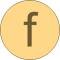# smoothStep

Approximation of a general step, such that the characteristic is continuous and differentiable# Information

This information is part of the Modelica Standard Library maintained by the Modelica Association.

This function is used to approximate the equation

```    y = if x > 0 then y1 else y2;
```

by a smooth characteristic, so that the expression is continuous and differentiable:

```   y = smooth(1, if x >  x_small then y1 else
if x < -x_small then y2 else f(y1, y2));
```

In the region -x_small < x < x_small a 2nd order polynomial is used for a smooth transition from y1 to y2.

If mass fractions X[:] are approximated with this function then this can be performed for all nX mass fractions, instead of applying it for nX-1 mass fractions and computing the last one by the mass fraction constraint sum(X)=1. The reason is that the approximating function has the property that sum(X) = 1, provided sum(X_a) = sum(X_b) = 1 (and y1=X_a[i], y2=X_b[i]). This can be shown by evaluating the approximating function in the abs(x) < x_small region (otherwise X is either X_a or X_b):

```    X  = smoothStep(x, X_a , X_b , x_small);
X  = smoothStep(x, X_a , X_b , x_small);
...
X[nX] = smoothStep(x, X_a[nX], X_b[nX], x_small);
```

or

```    X  = c*(X_a  - X_b)  + (X_a  + X_b)/2
X  = c*(X_a  - X_b)  + (X_a  + X_b)/2;
...
X[nX] = c*(X_a[nX] - X_b[nX]) + (X_a[nX] + X_b[nX])/2;
c     = (x/x_small)*((x/x_small)^2 - 3)/4
```

Summing all mass fractions together results in

```    sum(X) = c*(sum(X_a) - sum(X_b)) + (sum(X_a) + sum(X_b))/2
= c*(1 - 1) + (1 + 1)/2
= 1
```

# Syntax

y = smoothStep(x, y1, y2, x_small)

# Inputs (4)

x Type: Real Description: Abscissa value Type: Real Description: Ordinate value for x > 0 Type: Real Description: Ordinate value for x < 0 Default Value: 1e-5 Type: Real Description: Approximation of step for -x_small <= x <= x_small; x_small > 0 required

# Outputs (1)

y Type: Real Description: Ordinate value to approximate y = if x > 0 then y1 else y2